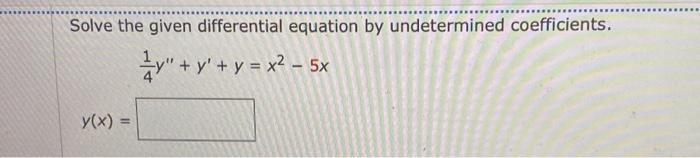Home / Expert Answers / Calculus / solve-the-given-differential-equation-by-undetermined-coefficients-41y-y-y-x25xy-x-pa566

# (Solved): Solve the given differential equation by undetermined coefficients. 41y+y+y=x25xy(x) ...Solve the given differential equation by undetermined coefficients.

We have an Answer from Expert